# Using Linear Variable Filters with Lasers

This article covers an example application of Linear Variable Filters from DELTA, using a laser beam as the light source. In this experiment, the effect of laser beam divergence on the spectral curves of the Linear Variable Filters is investigated.

The laser used was an NKT Photonics SuperK, with a beam divergence smaller than 5 mrad (0.29º) and a beam diameter of 1mm in VIS mode, 2mm at 1000nm wavelength and 3mm at 2000nm wavelength. the filter system included a linear variable LWP filter and a linear variable SWP filter in series.

• The beam diameter is assumed to be the same on both filters

• The spot size on the linear variable filter is given by the diameter of the area containing 1 – 1/e2 = 86.5% of the energy in the laser beam

• Beam divergence is not taken into account

• The intensity distribution in the laser beam from a super continuum light-source is considered to be purely Gaussian

• The light beam is assumed to be normal to the surface of the filters. In practice, the filters must be tilted by around 5 degrees to make a beam dump, which produces a slightly elliptical spot. Effects of this tilt on polarization are insignificant, and will also not be taken into account.

## Investigating the Light-Spot

The calculations take into consideration the differences in the thickness of the layers in the coatings in the length direction and normal to that on the substrate.

In a specific section, the spectral performance of the linear variable filter is considered to be equal to an analogous section on a line along the central part of the filter (x-axis).

The portion of the x-axis where the spot exist is divided into 97 parts of equal size. The total spot is divided into millions of tiny sections by the design software. Each of these parts makes a weighted contribution to one of the 97 parts produced on the x-axis.

Centring the laser spot at the X-axis at 60% of the total length of the coating on the filter, a 1-mm diameter spot gives the calculated weighting along the x-axis (Figure 1). Figure 2 depicts the corresponding plots for a diameter of the laser spot of 2mm and 3mm.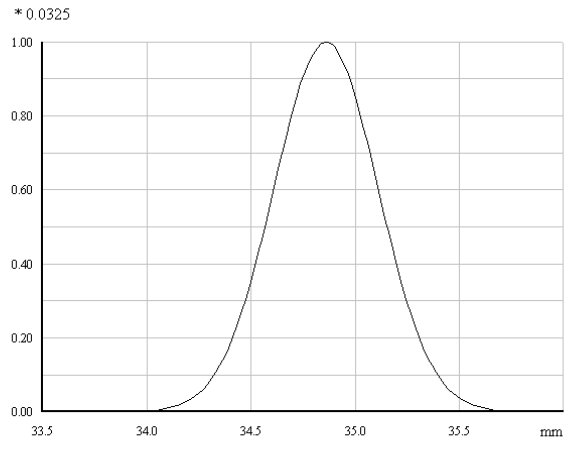Figure 1. Centring a laser spot with a spot diameter of 1mm at 60% of the full length of the coating on a 15mm x 60mm substrate, gives this weighted power distribution at the x- axis.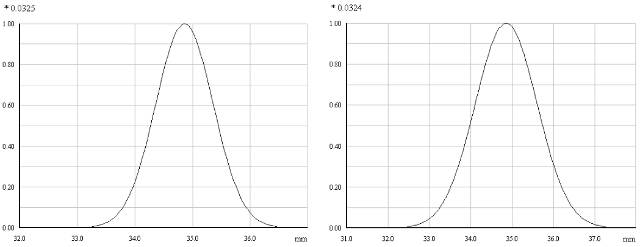Figure 2. Plots of the derived weighted power distributions along the central x- axis, for spot-diameters of 2mm and 3mm.

## Predicted Transmission Curves

The Linear variable SWP filter matches with the present product LVSP [300 - 850]nm LF102155, while the linear variable LWP filter matches with the present product LVLP [301-845]nm LF102064.

It is important to slightly offset the position along the x-axis on the linear variable LWP filter with regard to the position along the x-axis on the linear variable SWP filter to form a bandpass. The position along the X-axis will be referred in the form of a factor XL running from 0 to 1.

## Forming a Band Peaking at 630nm

Let us assume that XL is 0.6 for the SWP filter and 0.56 for the LWP filter, relating to a mechanical offset of approximately 58.1mm x 0.04 = 2.3mm. The best possible result for no spot-size and pure collimated light is shown in Figure 3.

In future, it may be possible to make filters that allow even steeper edges than this. However, in a real system the light spot has a final size.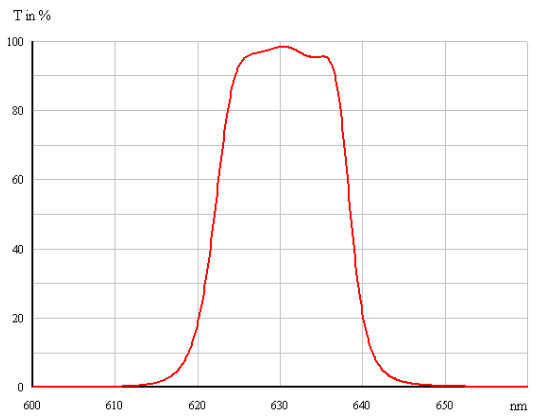Figure 3. The best possible result for No Spot size at the Linear variable filter LVSP [300 – 850]nm (XL =0.6) and the linear variable filter LVLP [301 – 845]nm (XL =0.56).

We will now find out what happens when the same set of filters is illuminated by a Gaussian shaped laser beam with a final diameter. Figure 4 displays the expected band formed as a function of the beam diameter.

The red curve belongs to the 1mm beam diameter, the blue curve to the 3mm beam diameter and the black curve to the 2mm beam diameter. The spot’s position on the two filters is described by XL(SWP) = 0.6 and XL(LWP) = 0.56. By increasing the bandwidth further, it would be possible to achieve the same peak transmission in all cases.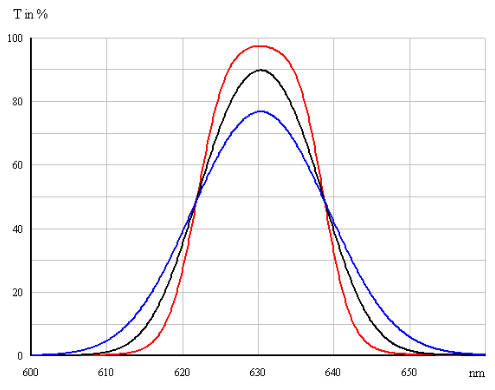Figure 4. Predicted bands formed by the linear variable LWP and SWP filters as function of the diameter of the laser-beam.

In case the diameter of the laser beam is only 1mm, it is practical to form a band with a bandwidth of below 20nm. However, the shape of the band is less box-shaped when compared to Figure 4, particularly at diameters larger than 1mm. As a result, the system does not get the optimum performance out of the filter system.

## Forming a Band Peaking at 433nm

Let us consider that XL is 0.18 and 0.22 in case of the LWP filter and SWP filter respectively, relating to a mechanical offset of approximately 58.1mm x 0.04 = 2.3mm. The predicted band formed as a function of the beam diameter is shown in Figure 5.

The red curve belongs to the 1mm beam diameter, the blue curve to the 3mm beam diameter and the black curve to the 2mm beam diameter. The spot’s position on the two filters is described by XL(SWP) = 0.22 and XL(LWP) = 0.18. By increasing the bandwidth further, it would be possible to achieve the same peak transmission in all cases.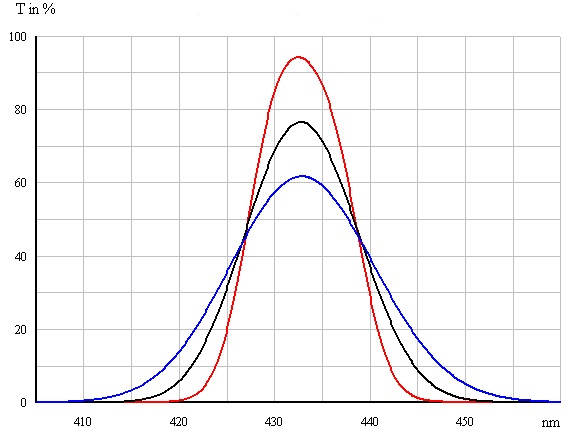Figure 5. Predicted bands formed by the linear variable LWP and SWP filters as function of the diameter of the laser beam.

If the diameter of the laser beam is only 1mm, it is reasonable to form a band with a bandwidth of less than 12nm. Comparison of Figures 4 and 5 shows that for a given peak transmission, the lowest bandwidth of the band increases proportionally with the centre wavelength.

## Adding Lens System to Reduce the Laser Beam Diameter

The diameter of the laser beam can be reduced by adding lenses. Although this would amplify the divergence angle, it would still be small - usually less than 1 degree, which does not cause any problems.

If a lens system is used to reduce the laser beam diameter by a factor of 3, the expected performance of the current filter set with 58.1mm coatings is predicted to be as shown in Figure 6.

It is assumed that by focusing the beam with a lens system the laser beam diameter is reduced by a factor of 3. The spot’s position on the two filters is described by XL(SWP) = 0.6 and XL(LWP) = 0.56.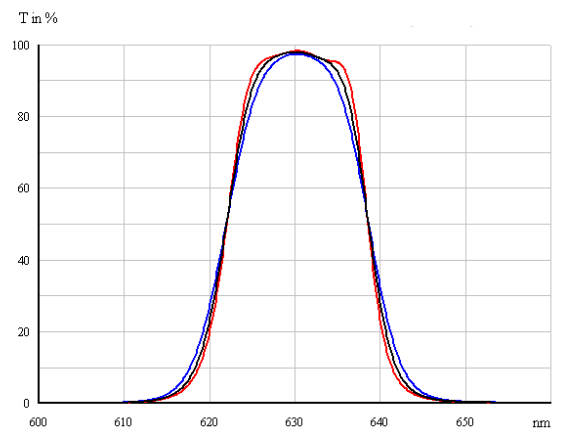Figure 6. Predicted bands formed by the linear variable LWP and SWP filters as function of the diameter of the laser-beam on the present filters. The red curve corresponds to a diameter of 0.33mm.

Comparison of Figures 3 and 4 shows that an even more box shaped band can be obtained and hence an even narrower bandwidth can be obtained by inserting a focussing lens in the wavelength selector. The expected result is close to the best possible result with the current coating designs.

## Conclusion

It is not possible to avoid lenses in most wavelength selectors based on linear variable filters, because a lens system is required to combine the filtered light into an optical fibre. However, a lensless system is possible if the light source is a laser.

In order to make the present 58.1mm-long filters (60mm substrates) work well, it is necessary to focus the light beam of the super continuum light source by a factor of 3.

The lenses can be avoided if the filters’ length is increased to 140mm or more. However, this would increase the price of the filters, and the system would not be able to leverage future enhancements of the spectral characteristics of the linear variable filters.This information has been sourced, reviewed and adapted from materials provided by DELTA Optical Thin Film.

## Citations

• APA

Delta Optical Thin Film A/S. (2019, October 23). Using Linear Variable Filters with Lasers. AZoOptics. Retrieved on November 22, 2019 from https://www.azooptics.com/Article.aspx?ArticleID=929.

• MLA

Delta Optical Thin Film A/S. "Using Linear Variable Filters with Lasers". AZoOptics. 22 November 2019. <https://www.azooptics.com/Article.aspx?ArticleID=929>.

• Chicago

Delta Optical Thin Film A/S. "Using Linear Variable Filters with Lasers". AZoOptics. https://www.azooptics.com/Article.aspx?ArticleID=929. (accessed November 22, 2019).

• Harvard

Delta Optical Thin Film A/S. 2019. Using Linear Variable Filters with Lasers. AZoOptics, viewed 22 November 2019, https://www.azooptics.com/Article.aspx?ArticleID=929.